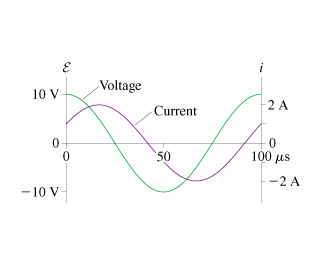# Problem: The figure shows voltage and current graphs for a series RLC circuit.What is the resistance R?

###### FREE Expert Solution

Current

$\overline{){\mathbf{I}}{\mathbf{=}}\frac{{\mathbf{\epsilon }}_{\mathbf{0}}}{\mathbf{Z}}}$

Impedance,

$\overline{){\mathbf{Z}}{\mathbf{=}}\sqrt{{\mathbf{R}}^{\mathbf{2}}\mathbf{+}{\mathbf{\left(}\mathbf{X}}^{}}}$L-XC)2

(1)

From the graph, at t = 0, I =Imax / 2. Substituting in the current equation and solving for Φ:

Imax/2 = Imax cos Φ

1/2 = cos Φ

Φ = 60°###### Problem Details

The figure shows voltage and current graphs for a series RLC circuit.What is the resistance R?